# Trigonometric Identities

## Right Triangle

The Trigonometric Identities are equations that are true for Right Angled Triangles. (If it is not a Right Angled Triangle go to the Triangle Identities page.)

Each side of a right triangle has a name:Adjacent is always next to the angle

And Opposite is opposite the angle

We are soon going to be playing with all sorts of functions, but remember it all comes back to that simple triangle with:

• Angle θ
• Hypotenuse
• Opposite

## Sine, Cosine and Tangent

The three main functions in trigonometry are Sine, Cosine and Tangent.

They are just the length of one side divided by another

For a right triangle with an angle θ :Sine Function: sin(θ) = Opposite / Hypotenuse Cosine Function: cos(θ) = Adjacent / Hypotenuse Tangent Function: tan(θ) = Opposite / Adjacent

For a given angle θ each ratio stays the same
no matter how big or small the triangle is

When we divide Sine by Cosine we get:

So we can say:

tan(θ) = sin(θ)cos(θ)

That is our first Trigonometric Identity.

## Cosecant, Secant and CotangentCosecant Function: csc(θ) = Hypotenuse / Opposite Secant Function: sec(θ) = Hypotenuse / Adjacent Cotangent Function: cot(θ) = Adjacent / Opposite

### Example: when Opposite = 2 and Hypotenuse = 4 then

sin(θ) = 2/4, and csc(θ) = 4/2

Because of all that we can say:

sin(θ) = 1/csc(θ)

cos(θ) = 1/sec(θ)

tan(θ) = 1/cot(θ)

And the other way around:

csc(θ) = 1/sin(θ)

sec(θ) = 1/cos(θ)

cot(θ) = 1/tan(θ)

And we also have:

cot(θ) = cos(θ)/sin(θ)

## Pythagoras TheoremThe Pythagorean Theorem says that, in a right triangle, the square of a plus the square of b is equal to the square of c: a2 + b2 = c2

Dividing through by c2 gives

a2 c2 + b2 c2 = c2 c2

This can be simplified to:

( a c )2 + ( b c )2 = 1

Now, a/c is Opposite / Hypotenuse, which is sin(θ)

And b/c is Adjacent / Hypotenuse, which is cos(θ)

So (a/c)2 + (b/c)2 = 1 can also be written:

sin2 θ + cos2 θ = 1

Note:
• sin2 θ means to find the sine of θ, then square the result, and
• sin θ2 means to square θ, then do the sine function

### Example: 32°

Using 4 decimal places only:

• sin(32°) = 0.5299...
• cos(32°) = 0.8480...

Now let's calculate sin2 θ + cos2 θ:

0.52992 + 0.84802
= 0.2808... + 0.7191...
= 0.9999...

We get very close to 1 using only 4 decimal places. Try it on your calculator, you might get better results!

Related identities include:

sin2 θ = 1 − cos2 θ
cos2 θ = 1 − sin2 θ
tan2 θ + 1 = sec2 θ
tan2 θ = sec2 θ − 1
cot2 θ + 1 = csc2 θ
cot2 θ = csc2 θ − 1

## How Do You Remember Them?

The identities mentioned so far can be remembered
using one clever diagram called the Magic Hexagon: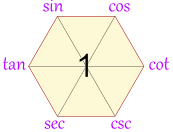## But Wait ... There is More!

There are many more identities ... here are some of the more useful ones:

### Opposite Angle Identities

sin(−θ) = −sin(θ)

cos(−θ) = cos(θ)

tan(−θ) = −tan(θ)

### Double Angle Identities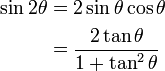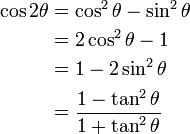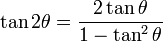### Half Angle Identities

Note that "±" means it may be either one, depending on the value of θ/2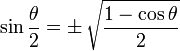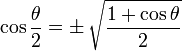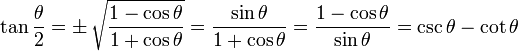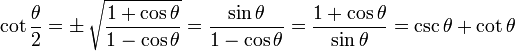### Angle Sum and Difference Identities

Note thatmeans you can use plus or minus, and themeans to use the opposite sign.

sin(AB) = sin(A)cos(B)cos(A)sin(B)

cos(AB) = cos(A)cos(B)sin(A)sin(B)

tan(AB) = tan(A)tan(B)1tan(A)tan(B)

cot(AB) = cot(A)cot(B)1cot(B)cot(A)

## Triangle Identities

There are also Triangle Identities which apply to all triangles (not just Right Angled Triangles)